# Asymptote

(diff) ← Older revision | Latest revision (diff) | Newer revision → (diff)

of a curve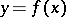with an infinite branch

A straight line the distance of which from the point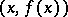on the curve tends to zero as the point moves along the branch of the curve to infinity. An asymptote can be vertical or inclined. The equation of a vertical asymptote is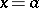, where(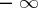) as(from one side) is satisfied. An inclined asymptote, with equation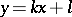, exists if and only if the limits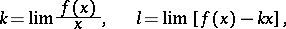exist as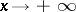(or as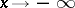).

Similar formulas are also obtained for parametrized (unbounded) curves in general parametric representation. In polar coordinates an asymptote of a curve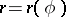, where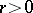, with slope angle, is defined by the condition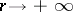as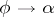. The distanceof this asymptote from the coordinate origin is calculated by the formula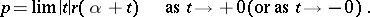If there exists a limit position of the tangent line to the infinite branch of the curve, this position is an asymptote. The converse is not always true. Thus, the curve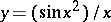has the asymptoteas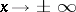, even though a limit position of the tangent line does not exist. Hyperbolas are the only second-order curves with asymptotes. The asymptotes of the hyperbola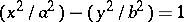are given by the equations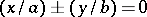. An inclined asymptote yields a simple (linear with respect to) asymptotic approximation of the function: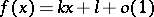as(or as).